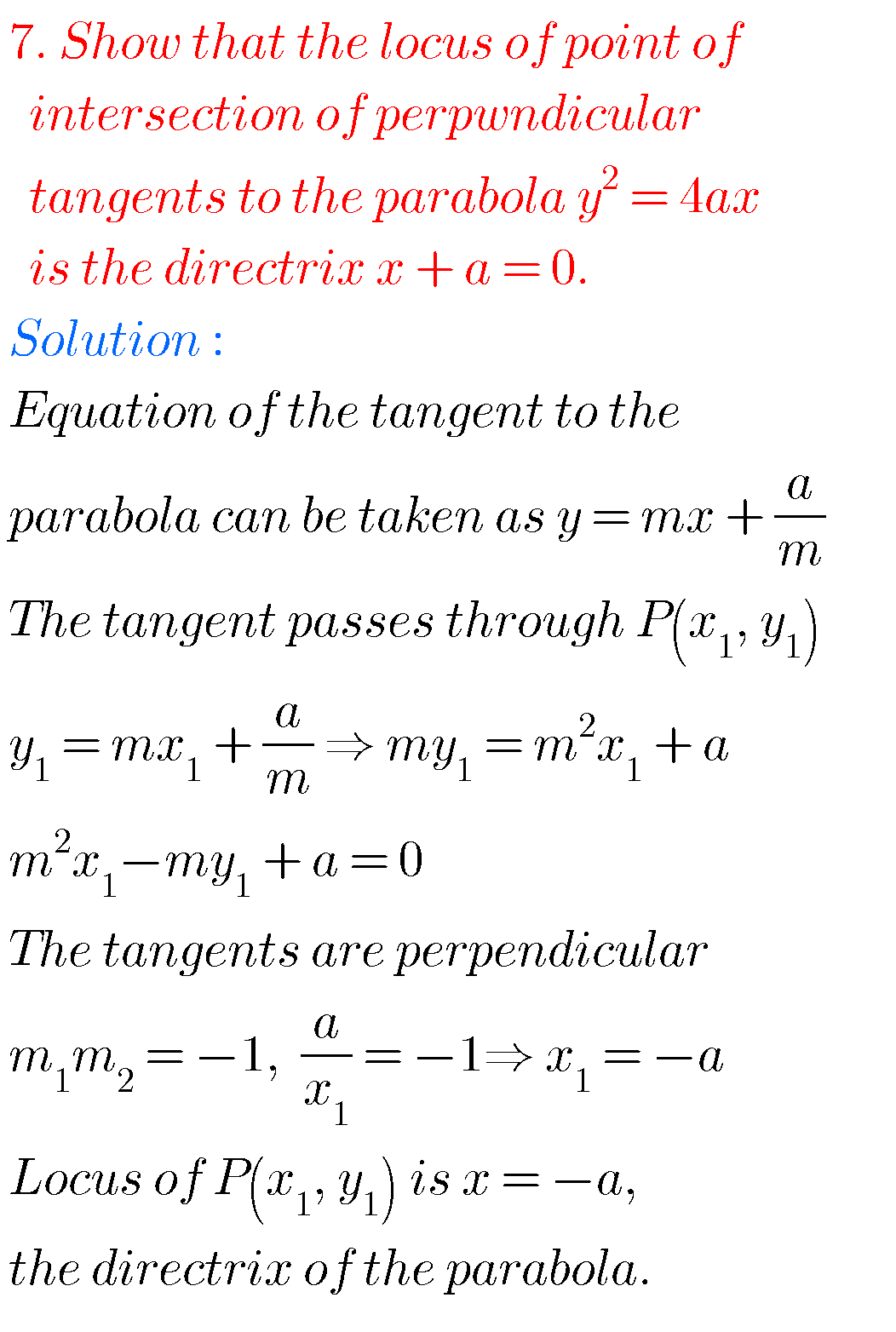## Intermediate maths solutions for Parabola

Intermediate maths solutions for Parabola Intermediate Mathematics IIB solutions for Parabola textbook exercise 3(a) and 3(b) are given. These solutions are very easy to understand. Study the textbook lesson very well. Then observe the example problems and solutions. Try them. Observe the given Inter maths 2b Parabola solutions. Try them in your own method. You …